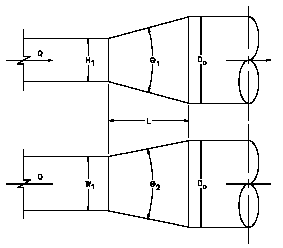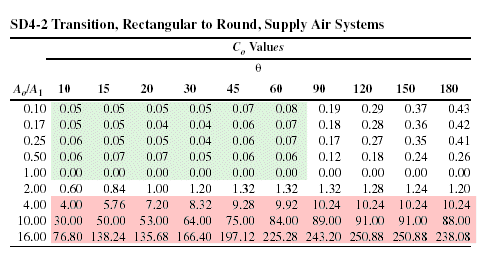# Square to Round Duct Fitting

`Author: B J Wernick PrEng BScEng`
`Date: 22 March, 2007`

The square to round is a common duct fitting that can be found in almost any duct system where rectangular ducting is connected to a tube-axial fan.ASHRAE has assigned the square to round fitting the number SD 4-2.  This stands for

S - Supply Duct

D - Diameter (Round Duct)

4 - Transition type

2 - 2nd in category

Refer to the ASHRAE Fundamentals for more details on the naming convention.The pressure drop is based on the fitting loss coefficient multiplied by the dynamic pressure.

Dynamic Pressure = ½ ρ V2

where

ρ = air density, typically 1.2 kg/m3 at sea level

V = air velocity, m/s

Fitting Pressure Drop = Co ·  ½ ρ V2

In a supply system, the air discharging from the fan could easily be in the order of 10m/s.  This means that the pressure drop at sea level would be 60xCo.  In a typical supply air system, a fan static pressure of 450 Pascals would not be unusual.  It would be impractical to select a single fitting with a pressure drop in excess of 1% of the total static.  This implies a loss coefficient of not more than 0.075.  Looking at the loss coefficient table, this means that we would exclude all fittings where Ao/Ai>=2 and θ >=90

The length L of the fitting would be based on the dimensions of the ducting.

### Example Calculation

Given a rectangular duct with the dimensions 915x700 going to a 700mm diameter fan connection with an airflow of 6.5 m3/s, calculate the pressure drop where L=400mm.

Ao = π D2/4 = 0.3848

A1 = H1 x W1 = 0.6405

Ao/A1 = 0.6

θ = 30°

From the loss coefficient table, Co = 0.06

Therefore the pressure drop is calculated as follows:

V = 10m/s

dP = Co ·  ½ ρ V2 = 3.6 Pascals

There is no reason why a shorter length should not be used but this would result in higher pressure drops that are not normally allowed for in selecting the fan.

### References

SAIRAC 2004 Technical Data Manual, Wernick B.J. Ed.

ASHRAE 2001 Fundamentals Handbook, Duct Design Chapter 34, pp 34.50